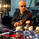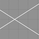3473 views
Request for Equinox, zigzag and fibs are extras :p
Open-source script

In true TradingView spirit, the author of this script has published it open-source, so traders can understand and verify it. Cheers to the author! You may use it for free, but reuse of this code in a publication is governed by House Rules. You can favorite it to use it on a chart.

Want to use this script on a chart?
```study("[RS]Equinox Timed Extremes V0", overlay=true)
ExtremeTimeFrame = input("240")
ExtremeTimeWindow = input(1)

TEH = security(tickerid, ExtremeTimeFrame, highest(ExtremeTimeWindow))
TEL = security(tickerid, ExtremeTimeFrame, lowest(ExtremeTimeWindow))

EH = plot(TEH, color=TEH!=TEH?na:black)
EL = plot(TEL, color=TEL!=TEL?na:black)
fill(EH, EL, color=gray, transp=75)
Hcon = high >= TEH
Lcon = low <= TEL

plotshape(Hcon, style=shape.triangledown, color=maroon, location=location.abovebar, text='[TEH]')
plotshape(Lcon, style=shape.triangleup, color=green, location=location.belowbar, text='[TEL]')
showFibLvls = input(true)
range = TEH-TEL

fib236 = TEL + range*0.236
fib382 = TEL + range*0.382
fib500 = TEL + range*0.500
fib618 = TEL + range*0.618
fib764 = TEL + range*0.764

plot(not showFibLvls ? na : fib236, color=fib236!=fib236?na:blue)
plot(not showFibLvls ? na : fib382, color=fib382!=fib382?na:teal)
plot(not showFibLvls ? na : fib500, color=fib500!=fib500?na:orange)
plot(not showFibLvls ? na : fib618, color=fib618!=fib618?na:teal)
plot(not showFibLvls ? na : fib764, color=fib764!=fib764?na:blue)

showZigzag = input(true)
zigzag = Hcon ? high : Lcon ? low : na
plot(not showZigzag ? na : zigzag, color=black, style=circles, linewidth=4, join=true)```